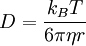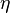Stokes-Einstein relationThis article is a 'stub' page, it has no, or next to no, content. It is here at the moment to help form part of the structure of SklogWiki. If you add sufficient material to this article then please remove the {{Stub-general}} template from this page.
The Stokes-Einstein relation, originally derived by William Sutherland (Ref. 1) but almost simultaneously published by Einstein (Ref. 2), states that, for a sphere of radius$R$ immersed in a fluid,$D=\frac{k_B T}{6\pi\eta r}$
where D is the diffusion constant,$k_B$ is the Boltzmann constant, T is the temperature and$\eta$ is the viscosity.